# Sigma and pi Orbitals

## Contents

### Molecular Orbital Theory

Molecular orbital theory was developed in the early part of the last century to help rationalize why bonds form and to explain the properties of molecules. In molecular orbital theory the atomic orbitals from each atom can overlap with those on other atoms. Since the atomic orbitals are wavefunctions and behave like waves it is possible for them to overlap in a constructive manner to form a bonding orbital.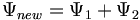$\Psi_{new} = \Psi_1 + \Psi_2\,\!$

Or the two functions can combine in a destructive manner.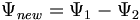$\Psi_{new} = \Psi_1 - \Psi_2\,\!$

This is sometimes referred to as Linear Combination of Atomic Orbitals (LCAO). When there is a destructive combination of the two waves there is a node between the two atoms where there is a zero probability of finding the electron. The first case is known as a bonding orbital and the latter case is known as an anti-bonding orbital.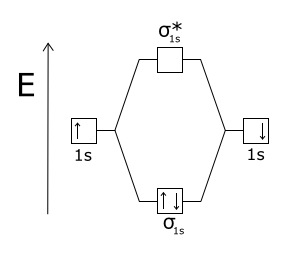Electrons from two atoms combine to form a σ bonding molecular orbital.

Note that the electron density is higher between the nuclei in the bonding molecular orbital and the that the orbital is stabilized, that is lower in energy relative to the two isolated hydrogren atoms. The sign of both wavefunctions is positive. There is a decreased electron density between the atoms in the antibonding molecular orbital and the orbital is destabilized, that is higher in energy than the two isolated hydrogen atoms. In very simple molecular orbital theory we treat the destabilization energy of the antibonding orbital as identical to the stabilization energy of the bonding orbital but in fact it is slightly more destabilized than the bond orbital is stabilized.

### Orbital Overlap

The amount of stabilization or destabilization is referred to a their orbital overlap. Mathematically the overlap is an integral of from negative infinity to positive infinity of a product of two wavefunctions over all space.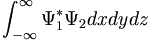$\int_{-\infty}^{\infty} \Psi_1^* \Psi_2 dxdydz\,\!$ = Overlap

The area under the curve for these functions is the orbital overlap.

If the s orbital is lined up exactly over the node of a p orbital you will end up with positive overlap on one side, and equal and opposite overlap on the other side. The orbital overlap when you integrate over all space will be identically zero. If you take two p orbitals that are oriented at 90 degrees the product is zero.

If you shift the s orbital a little bit one direction the overlap of the s orbital with one of the lobes will be non-zero. If you have two p orbitals at some angle you will have non-zero overlap. Or if a s orbital overlaps only on side of a the p orbital you would get some orbital overlap.

The two p orbitals that are aligned with the same sign will have the maxiumum orbital overlap and the maxium bonding interaction. If they are overlapping with opposite signs you have the maximum anti-bonding interaction. If you rotate them orthogonally they will be neither bonding or nor antibonding.

### p-p σ Bonding

Two p orbitals can combine contructively or destructively. In constructive situation you have a lower energy bonding orbital. If they align destructively you have the higher energy antibonding orbital. In a σ bond, if you look down the axis between the atoms bonded to each other, the orbital will appear cylindrically symmetric. In such cases the atoms at the end of the bonds can rotate without breaking the bond. This is the case from the bonds between the s orbitals in hydrogen. P orbitals can overlap in two different ways. If they overlap head-on a σ bond is formed.

### p-p π Bonding

If two p-orbitals overlap constructively then a π bond is formed. If you look down the axis of a π bond the orbital will not appear cylindrically symmetrical. The top has one sign the bottom has the opposite sign. There will be a node in the plane of the bond. The overlap of the orbital depends on critically on the angle between the orbitals. If the orbitals are not exactly lined up, the bond will begin to break and at 90° there be no πbonding. The stabilization of two p orbitals forming a σ bond is greater than two p orbitals forming a π bonds because p and σ bonds have more orbital overlap. As a consequence σ bonds are more stabilized and stonger than π bonds.

### Effect of field on π electrons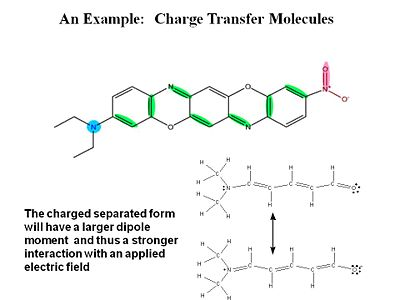The charge-separated form of this mole will react more to an applied field.

σ electrons have electron density between the nuclei so they are tightly bound and do not change position as much when exposed to an external field. π electrons have the electron density above and below of the plane of the nuclei. When you apply a field you change the distribution and induce a dipole.

For a typical molecule you go from a neutral ground state to a charge separated state by application of an electric field. Quantum -mechanically the electric field causes a mixing of these two states.

### Covalent bonding

When two atoms with duets or octets that have not been satisfied get within close proximity, they can interact in such a way as to create a bond by "sharing" electrons. Sharing of electrons can achieve a stable electron configuration corresponding to a noble gas configuration. On the right side of the diagram the hydrogen atoms are far apart and do not interact. As they move closer the system is stabilized by the formation of a bond through overlap of their orbitals and the lowering of the kinetic energy of the system. If the atoms get too close there will be an extremely strong nuclear - nuclear repulsion that greatly destabilizes the system. The correct balance between these interactions is found at the equilibrium bond length.

How does the potential energy surface for a hydrogen-hydrogen σ bond in the ground state (where the electrons are in the lower orbital) vs the excited states (ie where one electron is in an antibonding orbital)? These two states have a different energy dependence as a function of distance. A σ bonding combination initially becomes increasingly stabilized as you move from infinity until the point where the internuclear interaction (repulsion between protons) causes the energy to increase greatly. This point for hydrogen occurs at a distance of about .74 Å. The bond strength is 104 Kcal/mol, which is a very strong bond. The equilibrium constant for hydrogen radicals vs bond hydrogen would be 99999999.... favoring the bound state.

In the excited state the electrons are increasingly destabilized as the atoms get closer, the surface is repulsive. If you have two atoms infinitely far apart the system is neither stabilized nor destabilized. If you bring atoms to a correct geometry the ground state is stabilized. If you bring them too close you get a destabilized state. The excited state is always increasingly destabilized with decreasing distance.